## Introduction

The Rubik’s Cube is a relatively simple-looking puzzle from the outside and, sure enough, it is not that hard to actually solve one. This square tri-layered contraption, though innocent at first sight, actually possesses a deeper mathematical secret beneath the surface. While we won’t dive too deep into group theory, a rather complicated branch of mathematics, let’s really try to understand this puzzle to its roots!

## Construction of the Cube

Now, the Rubik’s Cube is composed of 6 centerpieces, 8 corner pieces, and 12 edge pieces, arranged in the form of a cube that is 3 pieces across any side. Each centerpiece has 1 face and is fixed into position, making it such that the face of the cube with a particular centerpiece is determined by the colour of the face of that centerpiece. Similarly, edge pieces have 2 faces and are lodged in between two centerpieces. Corner pieces have 3 faces and are lodged in between three centerpieces.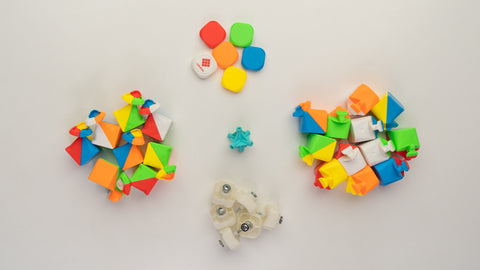All these put together comprise a basic 3x3x3 Rubik’s Cube, which can be seen in its solved state below.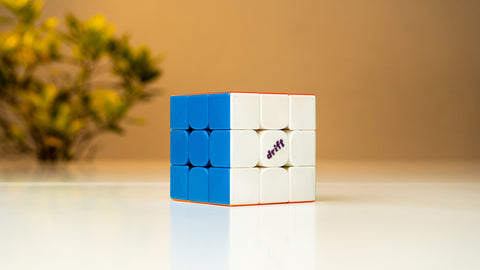## How large is 43 quintillion?

Now, it may come as a surprise that a cube with such a simple construction can actually be arranged in an enormous number of ways. In fact, this number has been found to be about 43 quintillion, which is 43 followed by 18 zeroes! One cannot even fathom how large this number is. It is larger than the total number of grains of sand on the Earth’s surface put together, and even the number of stars within our observable universe.Fascinating, isn’t it? But where did this massive number originate? How does one even arrive at such a large value? Certainly, this cannot be a guess and it definitely can’t be a number that a human can just ‘think of’.

## What is a permutation?

Without getting too mathematically intensive, a permutation on the Rubik’s cube is best understood as a ‘state’ of the puzzle. The easiest way to understand a state is just an arrangement of the various pieces (centers, edges, and corners) on the cube in the given fixed skeleton of a 3x3x3. By skeleton, I am referring to the essential structure of the cube, wherein it will always remain in the shape of a cube (a 3-dimensional solid with sides of equal length).So, a permutation is an arrangement of all the pieces on the cube within the shape of the cube. You may have heard of the steps OLL and PLL in the CFOP method to solve a cube. These stand for Orientation and Permutation of the Last Layer, respectively. Orientation refers to ensuring all the pieces are twisted correctly within their own spots (such as making the entire top layer the same colour). Permutation refers to moving these “correctly oriented” pieces around that top layer to their right spots.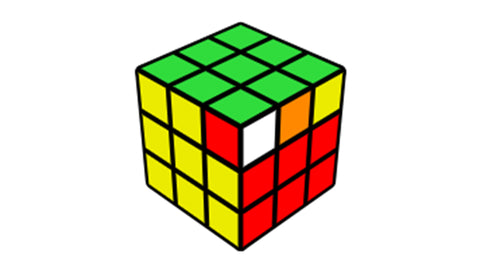On the Rubik’s Cube as a whole, the total number of permutations possible also includes a calculation of the orientation of each piece in the cube, since a change in any one orientation represents a different ‘state’ (defined before) of the cube, making it a permutation. Now, let’s dive into the actual determination of the total number of permutations possible on Rubik’s Cube.

## The Calculation

Before diving into this section, we need to remember something that I will term ‘dependency’. Let’s look at the following scenario: the orientation of a given corner/edge piece is completely determined by the orientations of the remaining corners/edges on the cube, in order to account for the states on the puzzle that aren’t normally possible. This can be referred to as the ‘dependence’ of that particular corner/edge on the remaining pieces.To further understand the states that aren’t possible, think about taking a solved cube and twisting just a single corner or flipping just a single edge. This would make the cube unsolvable since these are not positions that can be arrived at by simply turning the cube. Similarly, swapping just two edges (or two corners, which is the same idea on the whole) can create what we sometimes call ‘parity’ on bigger cubes, and also represents cases that are not possible on a 3x3x3 Rubik’s Cube.

Now that we completely understand what’s going on and what needs to be considered, let us jump into the calculation.

### Part 1: The Corners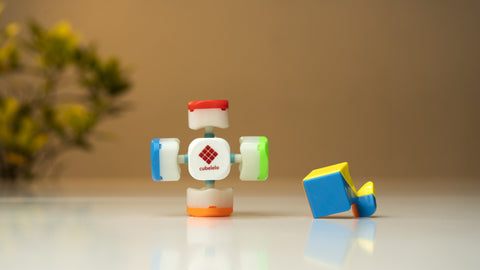There are 8 corners on a cube, which means the number of ways to arrange all the corner pieces (irrespective of orientation) into the cube is 8!.

Note- Factorial (!) represents the product of all numbers from the given number down to 1.

Why is it 8 factorial? Well, think of this. When assembling just the corners, you have 8 choices for the first corner’s position, 7 choices for the next, 6 for the next, and so on… Therefore, the total number of choices available to arrange the corners is: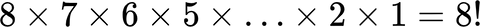Now, to account for orientation, each corner can be oriented in 3 states (since there are 3 faces on each corner). This is true for all corners except the last corner. By virtue of the ‘dependency’ we discussed, the last corner’s orientation is determined by those of the remaining 7. This means that every corner has 3 possible states while the last one has only 1. Thus, accounting for orientation, we get: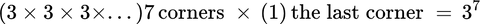Thus, the final number of possible states for the corners is:### Part 2: The EdgesThere are 12 edges on the cube, which means that when looking for ways to arrange 12 edge pieces, we get the trend: 12 choices for the first edge, 11 for the next, 10 for the next, and so on… until 1 for the last. This gives us a total number of arrangements:

Finally, each edge has two possible orientations in any position, since each edge piece has two faces. Again, due to ‘dependency’, the last edge’s orientation is determined by the orientations of all other edges, and therefore, it has only 1 right state instead of 2. Thus, the total number of orientations of edges is: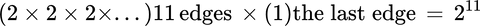The final number of possible states for the edges is:### Part 3: Parity

We haven’t considered the case when you have just a single corner/edge swap (between just two pieces). Note that an impossible corner swap is equivalent to an edge swap combined with a corner swap to make it possible. So, both these cases, be they corner or edge, are the same. But how can we include this problem in our calculations?Well, go back to the part where we were arranging the corners into places. We see that the positions of the last two corners are always fixed with respect to these corners. This means that while arranging the second last corner, instead of 2 choices, we actually have only 1 choice, since putting it in the other place would result in the final corner being in the wrong spot, representing parity!

Thus, the penultimate corner has only 1 spot, and so does the last! This is a new type of ‘dependency’ but still falls under the category of dependence. So, the number of arrangements available for the corners are now: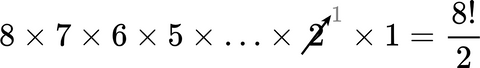### Part 4: The Result

Thus, combining these 3 parts of the calculation, we get our final number of possible states/permutations on the Rubik’s Cube to be: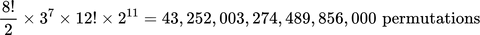That is 43 quintillion, 252 million, 3 trillion, 274 billion, 489 million, 856 thousand permutations on the Rubik’s Cube!

## Conclusion

So, we’ve finally arrived at the number for how many permutations are possible on the Rubik’s Cube and it was a relatively simple calculation if one understands how the process works. It is fascinating to think about how vast this number really is and no human can possibly comprehend how large this is!

A final thought I leave you with: God’s Number has been found to be 20. This means that any one of these 43 quintillion odd permutations can be solved in a mere 20 moves! That is all it takes to solve this monstrous number of configurations. How cool is that?Akshaansh Chilakapati

Akshaansh Chilakapati is a speedcuber from Hyderabad who specializes in big cubes. He started cubing when he was 15 and has 6 years of cubing experience. He loves to play sports, and music and has a passion for astrophysics. He has attended 23 competitions and won a total of 71 podiums with 19 gold medals. He was also ranked 7th in India for the overall Sum of Ranks (SOR).

All comments are moderated before being published

Top Selling Products

Drift 5-in-1 Beginner Kit
Sale price₹ 1,199
In stock
Drift 3M 3x3 (Magnetic)
Sale price₹ 549 Regular price₹ 599
8% off
In stock
Drift 3M PRO MagLev 3x3 (Magnetic)
Sale price₹ 1,149 Regular price₹ 1,299
12% off
In stock